# 改进粒子滤波的无人机三维航迹预测方法附Matlab代码

+关注继续查看

✅作者简介：热爱科研的Matlab仿真开发者，修心和技术同步精进，matlab项目合作可私信。

🍎个人主页：Matlab科研工作室

🍊个人信条：格物致知。

## ⛄ 内容介绍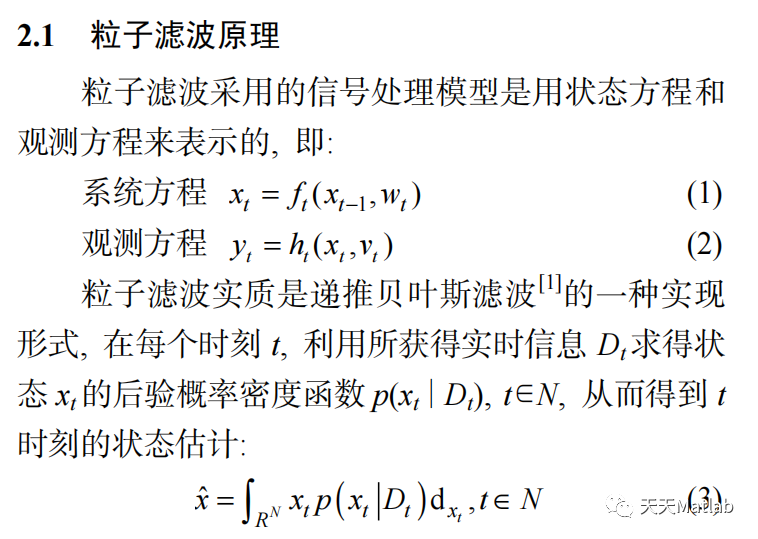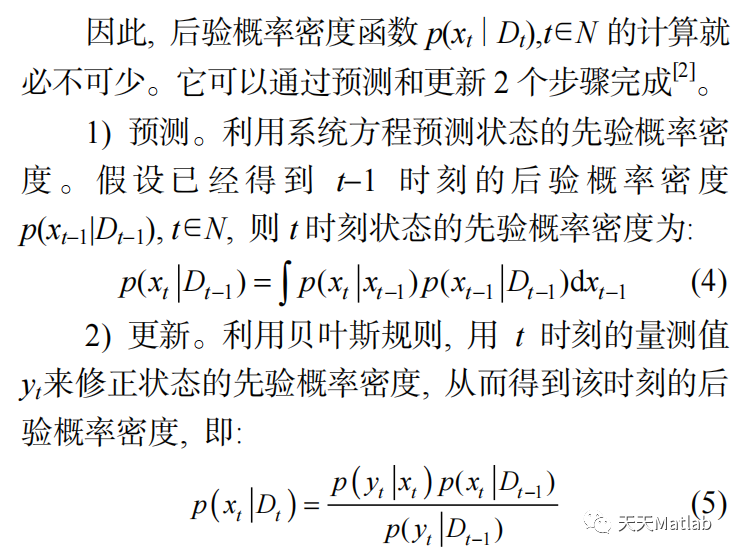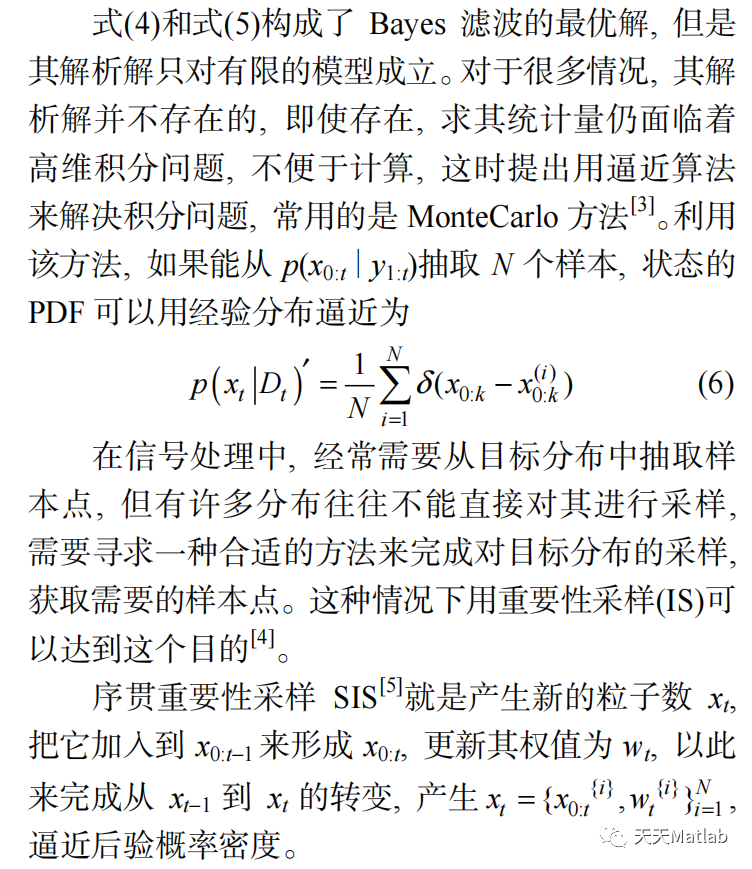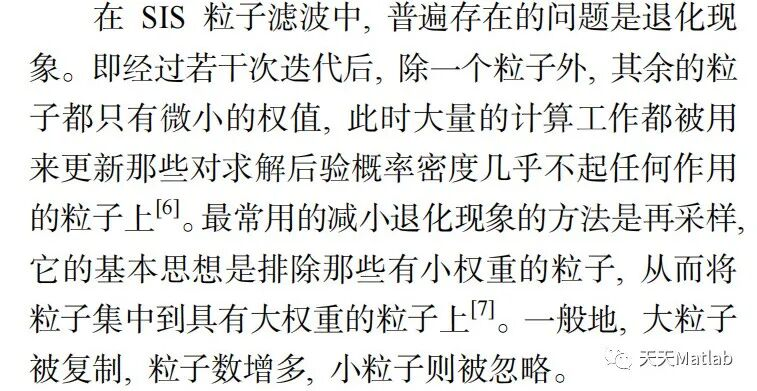## ⛄ 部分代码

%%%%%%%%%%%%%%%%%%%%%%%%%%%%%%%%%%%%%%%%%%%%%%%%%%%%%%%%%%%%%%%%%%%%%%%%%%

% 状态方程函数

function [y1,y2,y3,y4,y5,y6,y7,y8,y9] = ffun(x)

if nargin < 1

error('NoT enough inpuT argumenTs.');

end

T=0.5;

y1 = x(1)+T*x(4)+0.5*T^2*x(7);

y2 = x(2)+T*x(5)+0.5*T^2*x(8);

y3 = x(3)+T*x(6)+0.5*T^2*x(9);

y4 = x(4)+T*x(7);

y5 = x(5)+T*x(8);

y6 = x(6)+T*x(9);

y7 = x(7);

y8 = x(8);

y9 = x(9);

## ⛄ 运行结果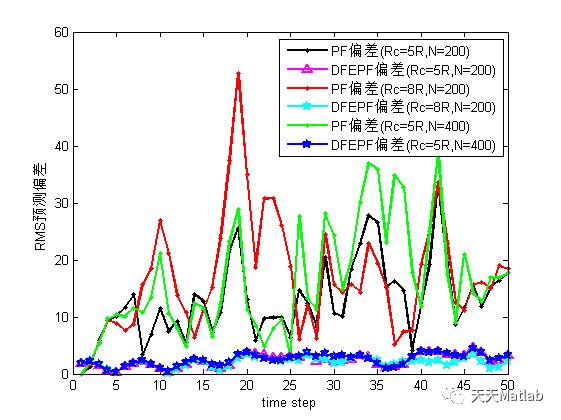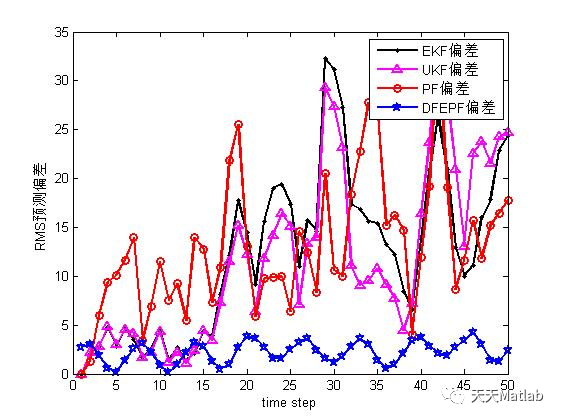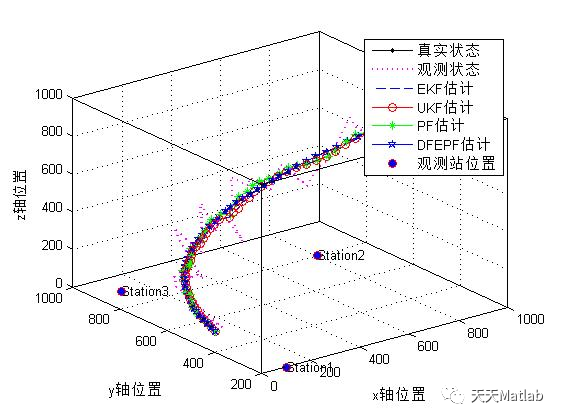## ⛄ 参考文献

曾润, 田杰, 江虹,等. 改进粒子滤波的无人机航迹预测方法[J]. 传感器与微系统, 2022, 41(5):4.

## ⛄ Matlab代码关注

##### ❤️ 关注我领取海量matlab电子书和数学建模资料0 0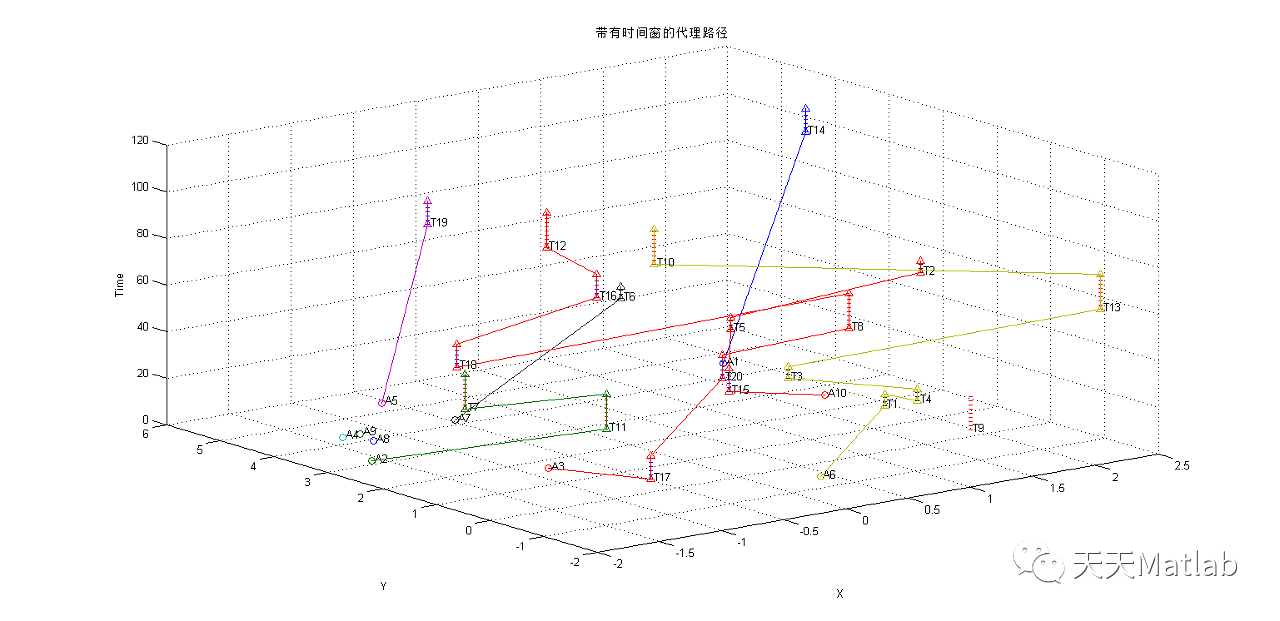【无人机分配】基于共识的捆绑算法CBBA实现带时间窗多无人机多任务调度附matlab代码
【无人机分配】基于共识的捆绑算法CBBA实现带时间窗多无人机多任务调度附matlab代码
0 0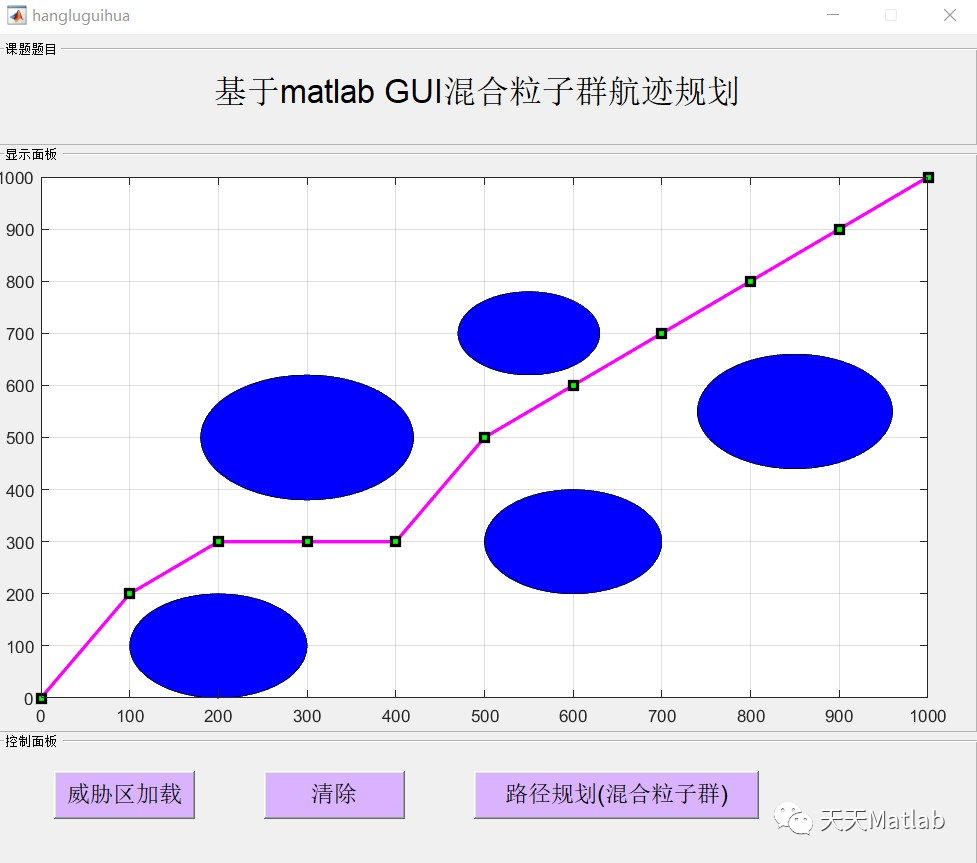【无人机】基于混合粒子群算法求解无人机航迹规划问题附Matlab代码
【无人机】基于混合粒子群算法求解无人机航迹规划问题附Matlab代码
0 0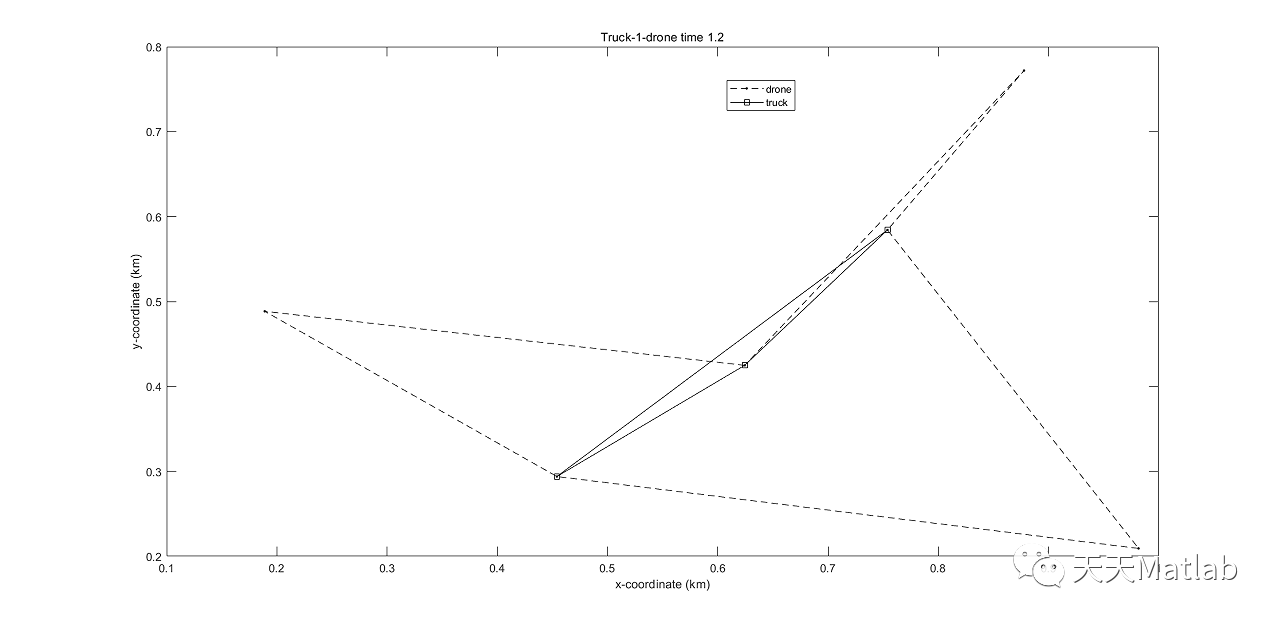【无人机】基于遗传算法的卡车结合两架无人机求解旅行推销员问题（D2TSP）附Matlab代码
【无人机】基于遗传算法的卡车结合两架无人机求解旅行推销员问题（D2TSP）附Matlab代码
0 0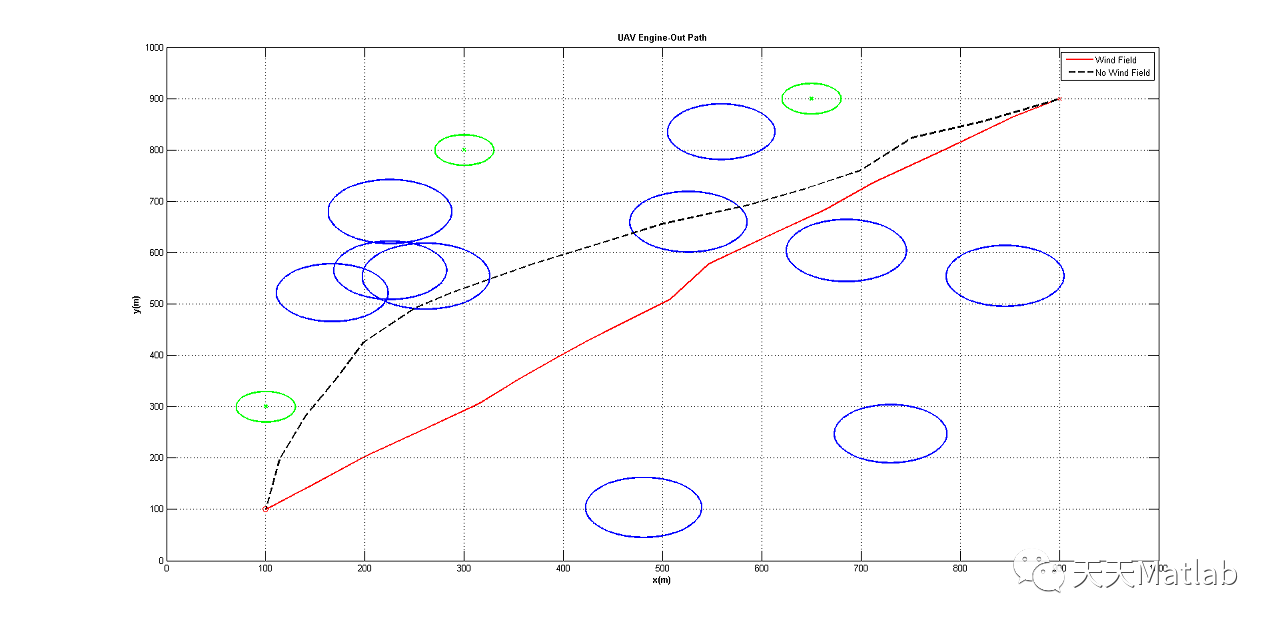【无人机】基于Fast行军树（FMT）求解无人机故障路径规划问题附matlab代码和论文
【无人机】基于Fast行军树（FMT）求解无人机故障路径规划问题附matlab代码和论文
0 0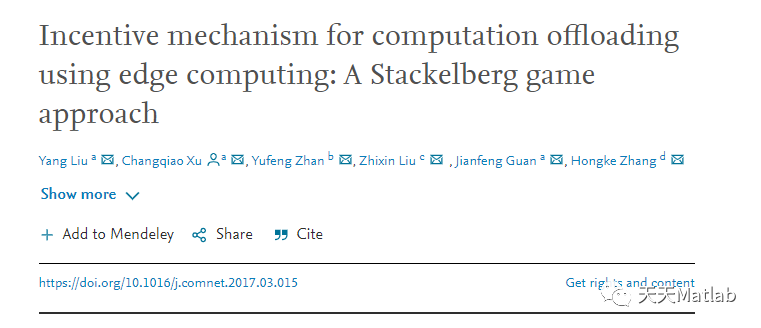0 0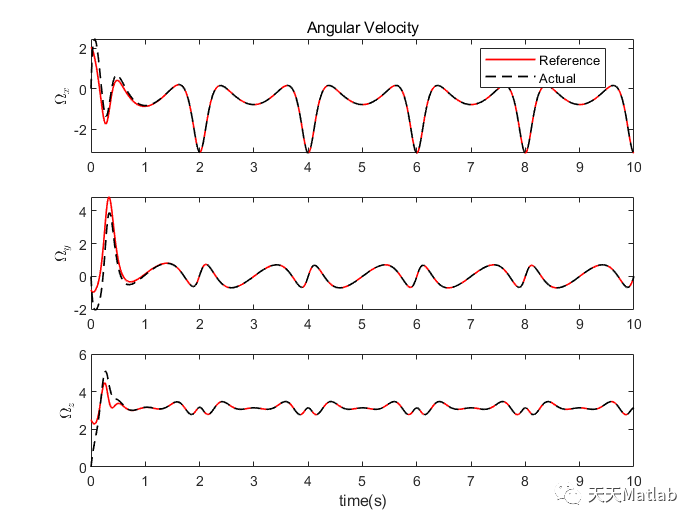【无人机】基于Matlab实现四旋翼无人机几何跟踪控制
【无人机】基于Matlab实现四旋翼无人机几何跟踪控制
0 0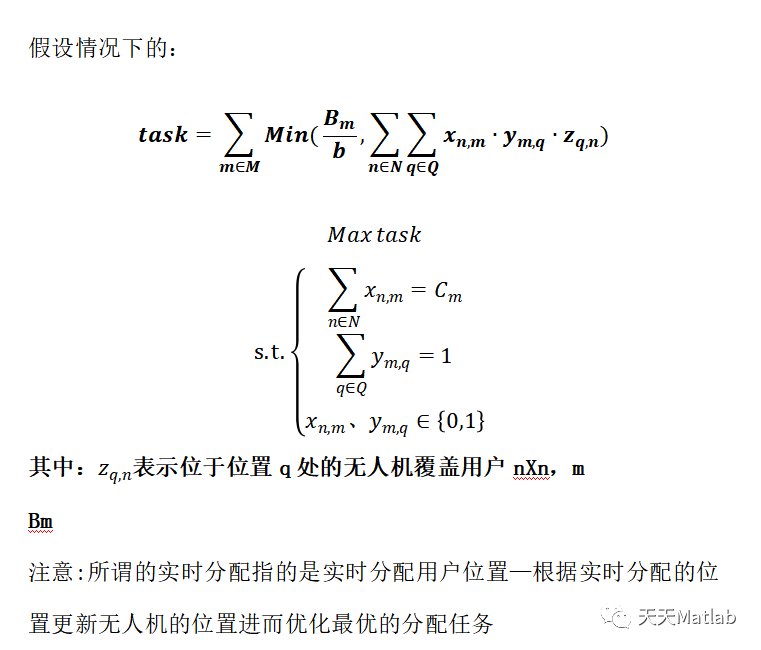【无人机分配】一种无人机实时最优任务分配模型附matlab代码
【无人机分配】一种无人机实时最优任务分配模型附matlab代码
0 0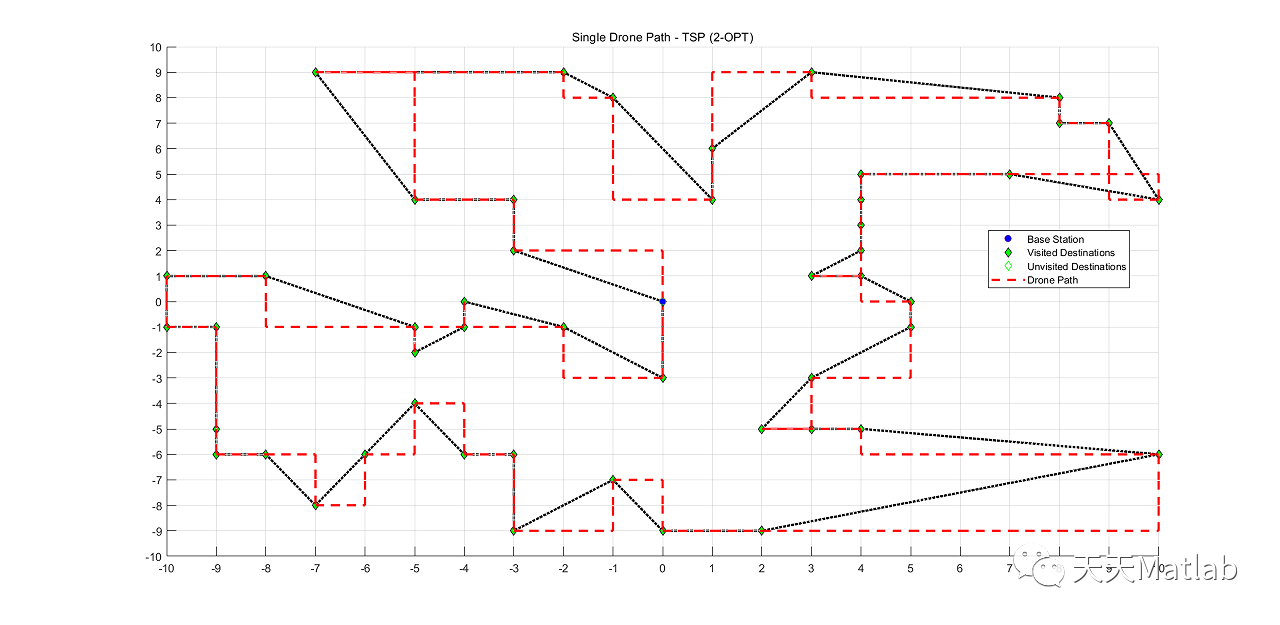0 0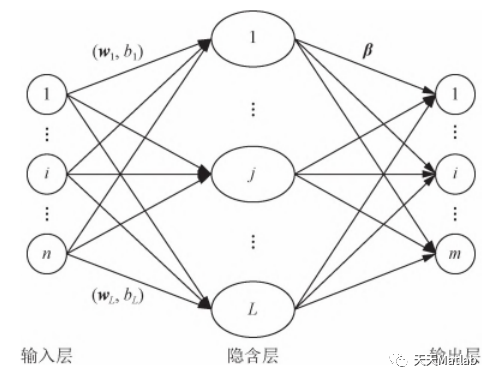【ELM时序预测】基于多尺度集成极限学习机实现寿命预测附matlab代码
【ELM时序预测】基于多尺度集成极限学习机实现寿命预测附matlab代码
0 0
Matlab科研工作室70613

【新人赛】工业蒸汽量预测建模算法代码开源分享合集1036